### Calculate the stretch for a linear spring given the spring constant and the applied force.

#### Example question:

How far will a linear spring with a constant of 630 N/m be stretched by a 140 N force?

#### Given:

spring constant: k = 630 N/m

force: F = 140 N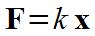Starting formula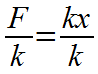Algebra: divide both sides by k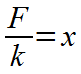Algebra: k's cancel on rightAlgebra: switch left and right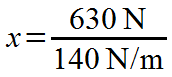Plug in numbers with units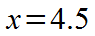Divide: 630/140 = 4.5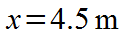Final result with significant figures and unit